JAVA, HTML5 & Chemistry Site

Other Writing

Last Update
19-Oct-2014

### Properties Estimation: For understanding of Surface Tension

2013.8.16

Lecture note of Dr. Hiroshi Yamamoto

Please refer to estimation method of surface tension

Surface Tension of liquids

Surface tension is a contractive tendency of the surface of a liquid that allows it to resist an external force.
The cohesive forces among liquid molecules are responsible for the phenomenon of surface tension. In the bulk of the liquid, each molecule is pulled equally in every direction by neighboring liquid molecules, resulting in a net force of zero. The molecules at the surface do not have other molecules on all sides of them and therefore are pulled inwards. This creates some internal pressure and forces liquid surfaces to contract to the minimal area.
(WikiPedia)

Can you sort these liquids according to surface tension at room temperature? (Carbon # 4 liquids)
Which index do you use to do that?

 Hcode Liquid 92 n-Butanol 255 Ehtyl ether 1060 n-Butylaldehyde 481 Methyl ethyl ketone 116 Butyronitrile 114 n-Butylic acid 328 Ethyl acetate 285 N,N-Dimethylacetoamide 260 Diethyl sulfide 91 1-Butanethiol 105 n-Butyl amine 252 Diethyl amine 81 1,3-Butadiene 1154 Decafluorobutane 152 1-Chlorobutane 3670 1-Bromobutane

We know that oil float on water. That is cause to lower density of oil than that of water. But we also know even aluminum metal float on water with the effect of Surface Tension. So we tend to think water-soluble liquids have high Surface Tension.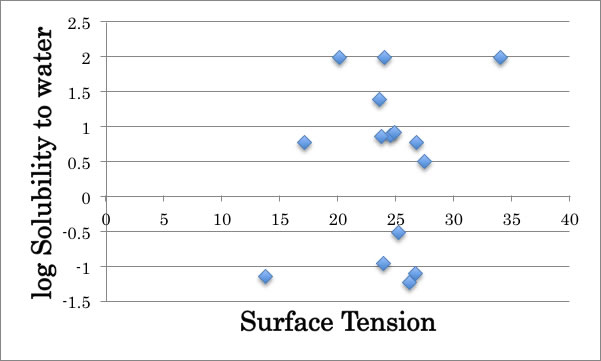But you can easily find out it is wrong if you plot Surface Tension to log Solubility to water.
You may use Dipole Moment or Dielectric Constant to assume Surface Tension.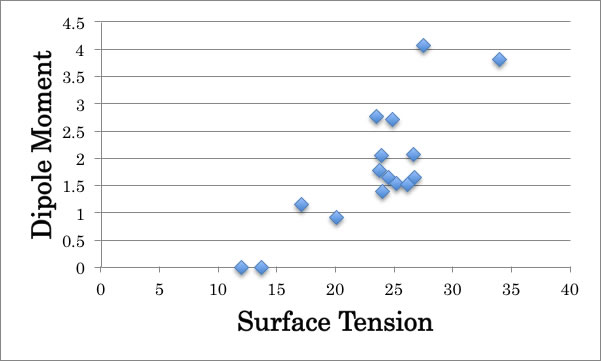##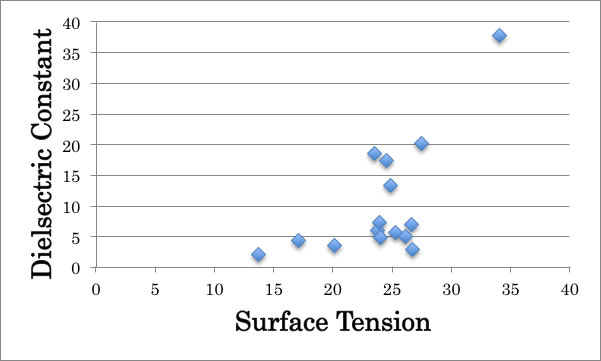It may work but not perfect.
There is no exact index, so we use very ambiguous word “Hydrophilic” and “Hydrophobic”. Hydrophilic compounds have high Surface tension and hydrophobic compounds have low Surface tension. Is that correct?
It seems OK if I plot temperature dependence of surface tension. Hydrophilic liquids such as Amide, Alcohol come to upper line and hydrophobic liquids such as Ether come to lower line. So it is not so bad idea, but problem is what the definition of that words.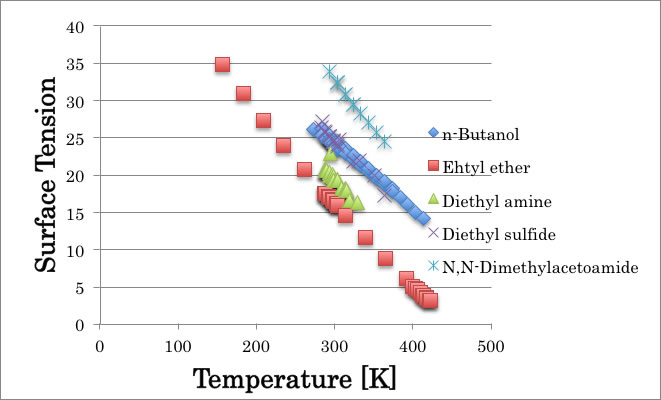Even though I admit Amin functional Group (NH) is hydrophobic, how we can explain the difference of Diethyl amine to Pyrrolidine or Pyrrole.

 Diethyl amine Pyrrolidine Pyrrole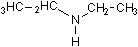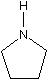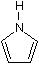γ=20.05 γ=29.65 γ=37.6

Ring NH is hydrophilic Functional Group, Ring NH next to double bond is much hydrophilic FG. How many type of NH should I determine?

 Ehtyl ether Tetrahydrofuran Furan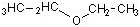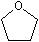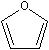γ=17.06 γ=27.2 γ=24.1 Diethyl sulfide Tetrahydrothiophene Thiophene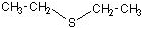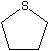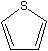γ=25.2 γ=35.8 γ=32.8

So I can’t determine functional group contribution to Surface Tension.

Even more, there is molecular shape effect.

 n-Butanol 2-Butanol i-Butanol t-Butanol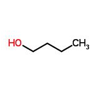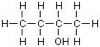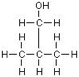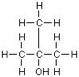γ=24.52 γ=23 γ=23 γ= 19.56

Beerbower (1971) propose very efficient scheme to predict Surface Tension.
γ=0.0715*MVol^1/3 [dD^2+0.632*(dP^2 + dH^2)]
dD, dP, dH are Hansen solubility parameters
This scheme is very good for large molecule, but if I apply for C4 molecules, result is not so good especially for all alcohol compounds.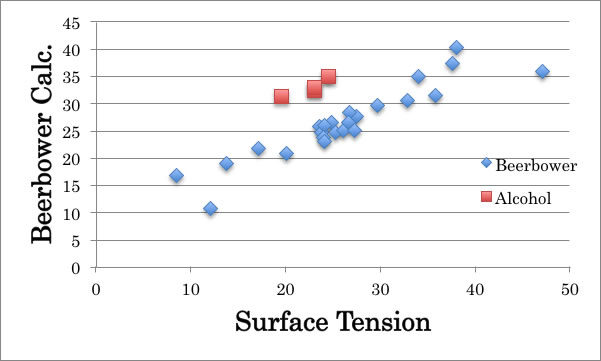So one of the best indexes to think of Surface Tension is Hansen Solubility Parameters (HSP). But all Alcohol becomes exception. You may think of this comes from hydrogen bonding effect, but n-butylic acid is not exception.

The original force of HSP is Heat of vaporization (Hv).
Total HSP = SQRT ((Hv-RT)/MVol)
(R: Gas Constant, MVol: Mol Volume)
Almost all case, the experimental measurement of Heat of Vaporization is done at boiling point. The result is most stable at there. But Hansen Solubility Parameters use Heat of Vaporization at 25℃.
We use the Watson rule to do this.

dHv2=dHv1((1-Tr2)/(1-Tr1))^0.375

(dHv1: Heat of vaporization at Boiling Point, Tr1 : Boiling Point / Critical Temperature, Tr2 : Reference Temperature / Critical Temperature )
So if you know Hv@BP BP and Tc, then you can calculate dHv2 : Heat of vaporization at Reference Temperature.
From where, 0.375 power index come?
How accurate this rule is?
I’d better explain these.
If I plot temperature to Heat of vaporization, I will get below chart.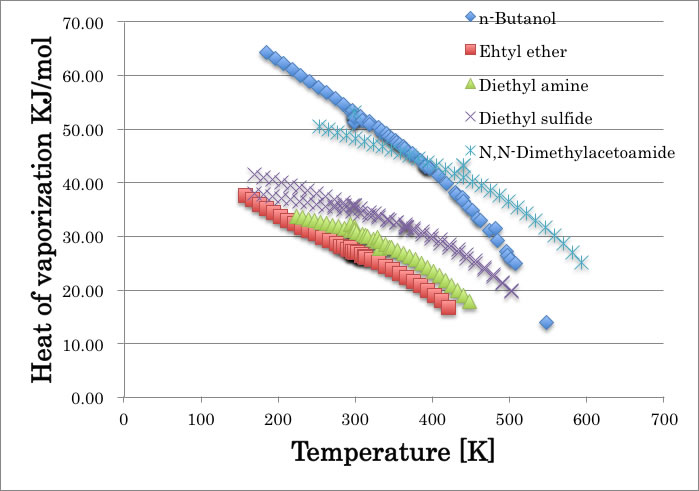The curves are very dependent to compounds. n-Butanol case, it loose hydrogen-bonding force as to elevate temperature, so Hv energy is sharply down. Other curves are almost parallel. And at critical temperature, all Hv become zero.
And what Watson rule said is change X-axis term temperature to (1-T/Tc).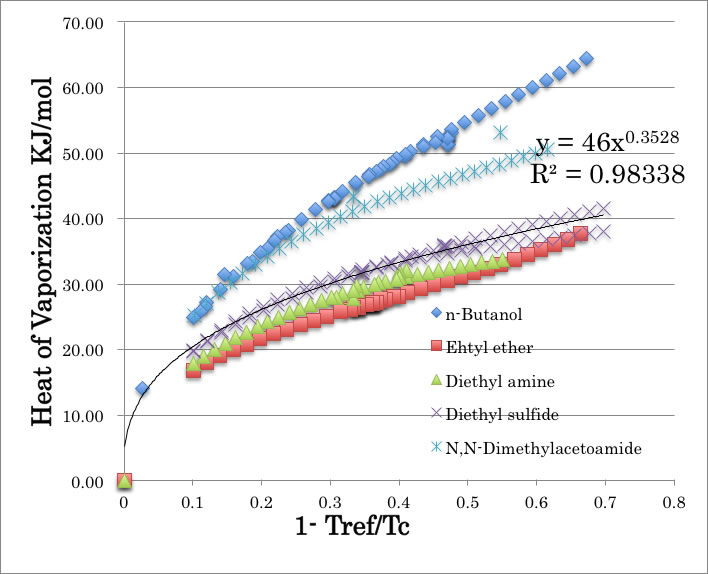And all of the compound’s curves become identical except hydrogen-bonding compounds.  So we can’t apply Watson rule to hydrogen-bonding compounds
And the curve fit, for example, diethyl sulfide’s curve power index become 0.3528. So, the 0.375 power index in Watson scheme is average of these curves (except hydrogen-bonding compounds).

Surface Tension case, it also become zero at Critical Temperature. So plotting with (1-Tref/Tc) is effective.Convert X-Axis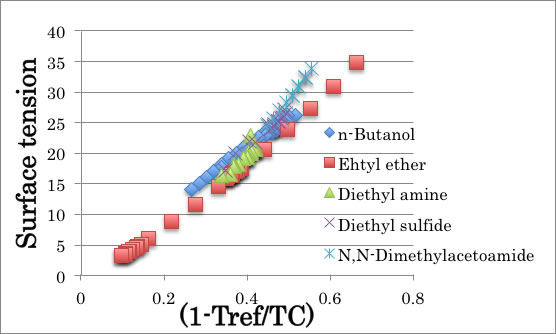Different from Heat of Vaporization, Hydrogen-bonding compounds are not became exception.
What this chart said is that Surface tension of liquids are determined by only Tc (Critical Temperature). The higher Surface tension means reference temperature is far from Tc. Fluorinated carbon has very low surface tension because Tc is low and near to room temperature. We always based Surface tension at 25℃, but if we based  (1-Tref/Tc), there is no difference “hydrophobic”, “hydrophilic” or “hydrogen-bonding” compounds.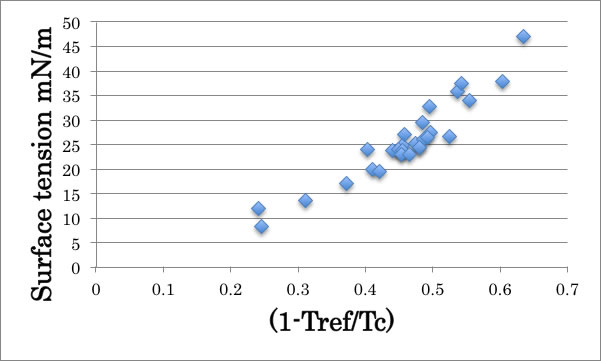I got the very good scheme to estimate surface tension for every kind of compounds at any temperature. This help to calculate Marangoni Number.

Now atom effect is canceled, I can think of shape effect.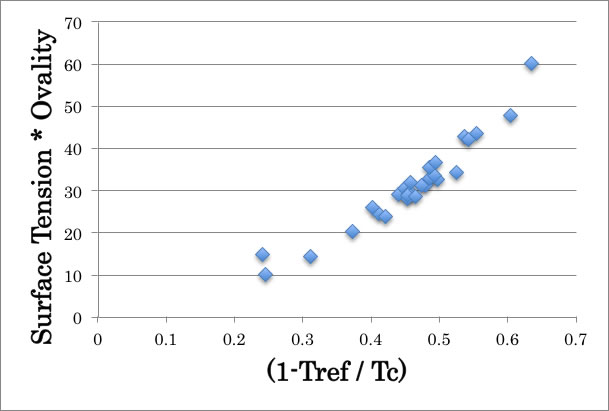This Ovality effect increases the correlation.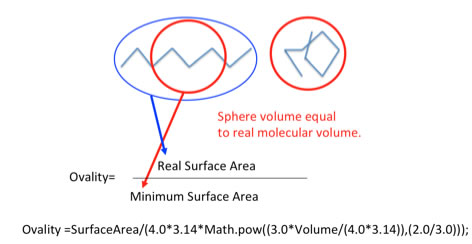In the Beerbower paper (1971), I found very interesting scheme.
d (ln γ)/dT = -4.0 * α
α: Thermal expansivity
This scheme is derived from Macleod – Sudgen scheme.
γ= (P*(ρ-d)/M)^4
P: parachor, ρ: density of liquid, d: density of Gas, M: molecular weight

Then, set density of Gas =0
γ= (P/V)^4
lnγ= 4*ln(P) – 4*ln(V)
If Parachor has no temperature dependency, the differential with temperature,
d (ln γ)/dT = -4.0 * d ln(V)/dT

If I use temperature, the curves are dependent to type of molecule, so I use (1 – Tref/Tc).

γ = 82.106* X^1.5069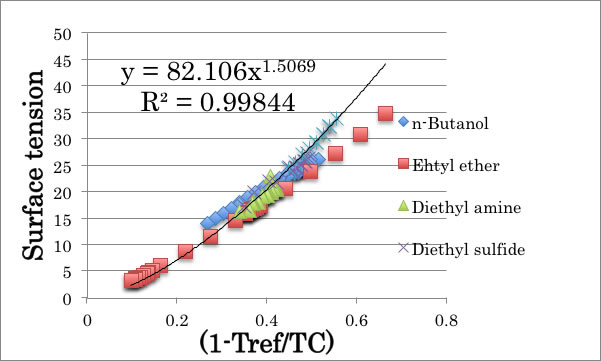Volume = 77.82 * X^-0.221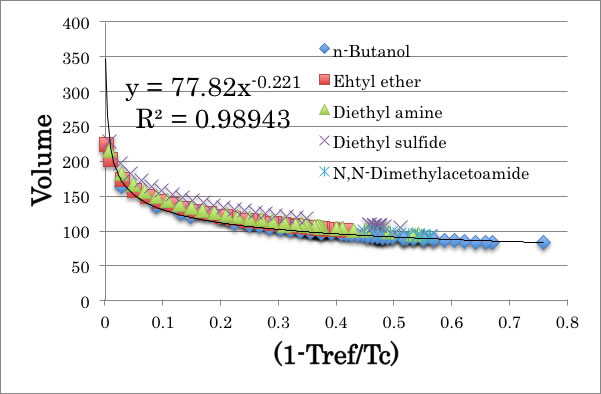82.106 and 77.82 are almost same, so from 2 curves, I got the equation.
ln γ = -7.0 * ln Volume + Const.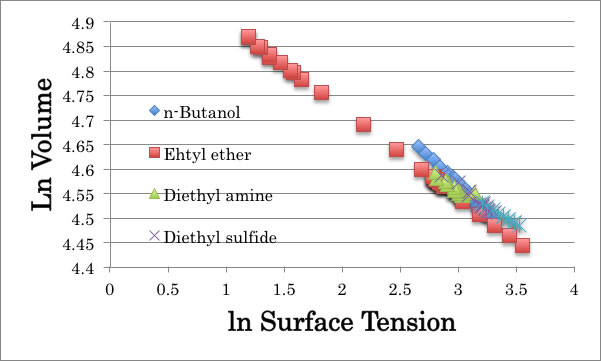And I got good agreement.

Macleod – Sudgen scheme, the temperature dependency is introduced only by density change by temperature.
γ= (P*(ρ-d)/M)^4
P: parachor, ρ: density of liquid, d: density of Gas, M: molecular weight

And this Parachor is temperature independent property. The Parachor and Molar Refraction[RD] has very good correlation.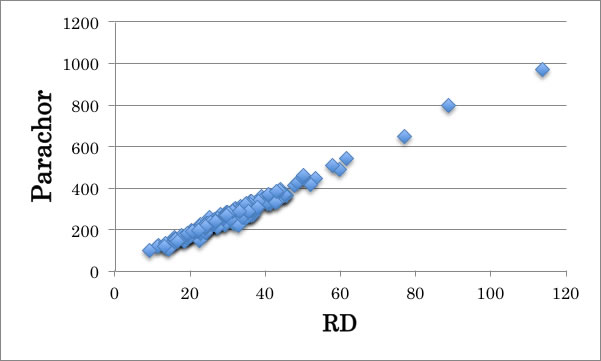We found that to estimate Molar Refraction[RD], HSP play very important role. Please refer to Estimation of Refractive Index article.

With all these results, I built Hansen type 4-dimensional surface tension estimation scheme at any temperature.

Butyronitrile example: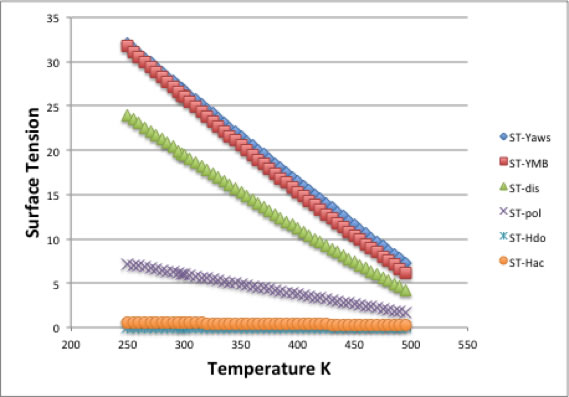n-Butanol example: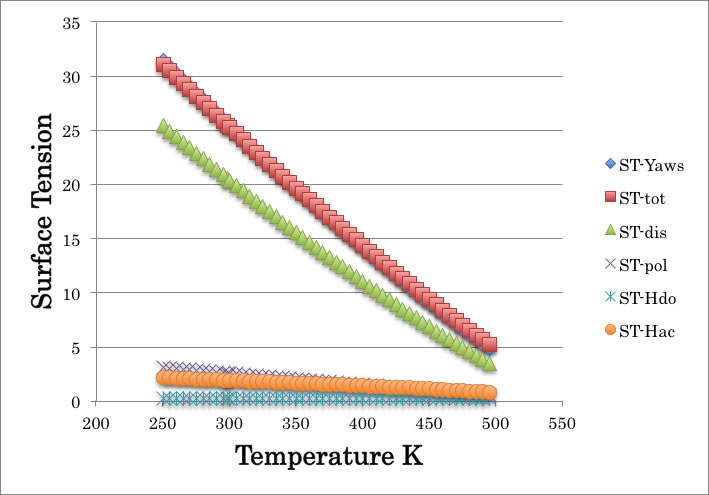Green line : ST-dis, dispersion term
Purple line: ST-pol, polarity term
Cyan line: ST-hdo, hydrogen-bonding donor term
Orange line: ST-hac, hydrogen-bonding acceptor term

The total surface tension become,
ST-tot (Red line) = ST-dis + ST-pol + ST-hdo + ST-hac

Hydrogen bonding terms become very low value even for alcohol because “hydrophobic” or “hydrophilic” nature plays nothing to surface tension. This is the great difference from Hansen Solubility parameters (HSP).

HSPiP user will soon available this new function